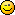# ETMods.net

N!tmod, a Wolfenstein: Enemy Territory Modification!

You are not logged in.

## Announcement

You can donate to help us keeping services online.## #1 31-Aug-12 20:32:28

Tasty
Member
Registered: 31-Aug-12
Posts: 3

### Howfair command

Hello, i'm new to lua scripting and today i've tried to create a command that shows us how fair the teams are (designed for hide&seek).

``````function allie()
local maxclients = tonumber( et.trap_Cvar_Get( "sv_maxClients" ))
local AllieP = 0

for i=0, maxclients do -- number of players in game
if (tonumber(et.gentity_get(i, "sess.sessionTeam")) == 1) or (tonumber(et.gentity_get(i, "sess.sessionTeam")) == 2) then
numIngame = numIngame + 1;
end
end

for i=0, maxclients do -- number of Allies
if (tonumber(et.gentity_get(i, "sess.sessionTeam")) == 1) then
numAllies = numAllies + 1;
end
end

for i=0, maxclients do -- number of Axis
if (tonumber(et.gentity_get(i, "sess.sessionTeam")) == 2) then
numAxis = numAxis + 1;
end
end

if (numAxis ~= 0) then
AllieP = (1 - ((numAllies / numAxis) * (5 / 3))
end
return AllieP
end

function axis()
local maxclients = tonumber( et.trap_Cvar_Get( "sv_maxClients" ))
local AxisP = 0

for i=0, maxclients do -- number of players in game
if (tonumber(et.gentity_get(i, "sess.sessionTeam")) == 1) or (tonumber(et.gentity_get(i, "sess.sessionTeam")) == 2) then
numIngame = numIngame + 1;
end
end

for i=0, maxclients do -- number of Allies
if (tonumber(et.gentity_get(i, "sess.sessionTeam")) == 1) then
numAllies = numAllies + 1;
end
end

for i=0, maxclients do -- number of Axis
if (tonumber(et.gentity_get(i, "sess.sessionTeam")) == 2) then
numAxis = numAxis + 1;
end
end

if (numAxis ~= 0) then
AxisP = ((numAllies / numAxis) * (5 / 3))
end
return AxisP
end

function et_ClientCommand(clientNum, command)

if (command == string.lower("howfair")) then
AllieT = allie()
AxisT = axis()
et.G_Print(-1 , "print \"^7Allies:^q %d ^7Axis:^q  %d\n\"", AllieT, AxisT)
return 1
end
end``````

I guess there are many errors, but that would be great if someone can explain me what is wrong their, Thanks in advance.

Last edited by Tasty (03-Sep-12 22:05:42)

Offline

## #2 06-Sep-12 17:54:28

ailmanki
BETA Tester
Registered: 08-Jun-12
Posts: 42
Website

### Re: Howfair command

Quite some redundancy..
maybe you would have just needed to use

``et.trap_SendServerCommand(-1,"print \"Hello Client\n\"") -- printout our text to the console``

``et.G_Print(-1 , "print \ ......) ``

not sure though, have not tested it.

``````function count()
local maxclients = tonumber( et.trap_Cvar_Get( "sv_maxClients" ))
local AxisP = 0
local numIngame = 0
local numAllies = 0
local numAxis = 0
for i=0, maxclients do -- number of players in game
clientteam = tonumber(et.gentity_get(i, "sess.sessionTeam"))
if (clientteam == 1) or (clientteam == 2) then
numIngame = numIngame + 1;
elseif (clientteam == 1) then
numAllies = numAllies + 1;
elseif (clientteam== 2) then
numAxis = numAxis + 1;
end
end

if (numAxis ~= 0) then
AxisP = ((numAllies / numAxis) * (5 / 3))
end
return AxisP
end

function et_ClientCommand(clientNum, command)
if (command == string.lower("howfair")) then
local AxisT
local AllieT = 0
AxisT = count()
if (AxisT ~= 0) then
AllieT = (1 - AxisP)
end
et.trap_SendServerCommand(-1,"print \"^7Allies:^q " + AllieT + " ^7Axis:^q " + AxisT + "\n\"")
return 1
end
end``````

Last edited by ailmanki (06-Sep-12 17:56:06)

Offline

## #3 06-Sep-12 20:10:06

Tasty
Member
Registered: 31-Aug-12
Posts: 3

### Re: Howfair command

it didn't work but it helped me a lothere is the final code (working)

``````function round(number, precision)
return math.floor(number*math.pow(10,precision)+0.5) / math.pow(10,precision)
end

function count()
local maxclients = tonumber( et.trap_Cvar_Get( "sv_maxClients" ))
local AxisP = 0
local numAllies = 0
local numAxis = 0
local num = 1

for i=0, maxclients do
clientteam = tonumber(et.gentity_get(i, "sess.sessionTeam"))
if (clientteam == 1) then -- Axis
numAxis = numAxis + 1
elseif (clientteam == 2) then -- Allies
numAllies = numAllies + 1
end
end

if (numAllies ~= 0) then
AxisP = ((numAxis/numAllies) * (1/6))
num = round(AxisP, 2)
end

return num
end

function et_ClientCommand(clientNum, command)
local AxisT = 0
local AllieT = 0

if (command == string.lower("howfair")) then
AxisT = count()
AllieT = (1 - AxisT)
et.trap_SendServerCommand(-1,"chat \"                 ^p[^7Allies:^q ".. AllieT .." ^p| ^7Axis:^q ".. AxisT .."^p]\n\"")
return 1
end
end``````

Last edited by Tasty (14-Sep-12 12:46:48)

Offline